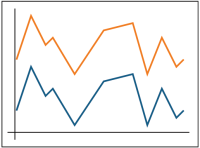## 5.12 Correlation TestsCorrelation tests can be used to assess whether two groundwater variables have a linear relationship with each other. Correlation tests may be used to evaluate both positive (when one variable increases, the other variable increases) and negative (when one variable increases, the other variable decreases) correlations. An example of a positive correlationAn estimate of the degree to which two sets of variables vary together, with no distinction between dependent and independent variables (USEPA 2013b). would be an observation that chemical concentrations in a well increase when water levels in the well increase. An example of a negative correlation would be an observed decrease in concentrations when the pumping rate for a groundwater extraction system is increased. These tests may also be used to test for monotonic trends or to compare trends.

### 5.12.1 Pearson Correlation Test

The parametricA statistical test that depends upon or assumes observations from a particular probability distribution or distributions (Unified Guidance). Pearson correlation test provides a measure of the linear association between two continuous variables. To conduct the test, correlation coefficients are calculated for each (x,y) pair, and the values of x and y are subsequently replaced with their ranks. Application of the test results in a correlation coefficient that ranges from -1 to 1. The sign of the coefficient indicates the direction of the relationship (that is, negative values imply an inverse relationship or a decreasing trend), and its absolute value indicates its strength, with larger (absolute) values indicating stronger linear relationships.

### 5.12.2 Spearman Rank Correlation Coefficient

The Spearman rank correlation test is essentially the nonparametricStatistical test that does not depend on knowledge of the distribution of the sampled population (Unified Guidance). version of the Pearson correlation coefficient test, and provides a measure of the linear association between two variables. Spearman’s rank correlation coefficient rho (ρ) is a nonparametric correlation coefficient that can be used to test for monotonic trends. To calculate the correlation coefficient ρ for any pair of variables x and y, each value of x is replaced with its rank R(x) and each corresponding value of y is replaced with its rank R(y). For concentrations sequentially measured over time (such as those, from a monitoring well), the x variable denotes time and R(x) is the sampling event order (R(x) = 1 for the first sampling event). The rank of the smallest concentration measurement is 1 (when it is not tied with other values).

Spearman’s ρ is similar to Pearson’s r that is calculated for the paired ranked results (1, R(y1)), (2, R(y2)), … (n, R(yn)) (for instance using Equation 3.5 in Chapter 3.5, Unified Guidance). Like the Pearson’s r, Spearman’s ρ ranges from -1 to 1 and can be tested to determine whether it is significantly different from zero; a positive value indicates an increasing trend and a negative value indicates a decreasing trend. The absolute value of the coefficient indicates its strength, with larger (absolute) values indicating stronger linear relationships.

When the sample size n is large (n > 20), the test statistic t = ρ (n- 2)½/(1 - ρ2)½ approximately follows the Student’s t distribution with n – 2 degree of freedom. To test whether there is a significant trend, the statistic t is compared with upper and lower percentiles of the Student’s t distribution. A large value of t (for example, greater than the 95th percentile of the Student’s t distribution with n-2 degree of freedom) suggests a significant increasing trend; a negative value (less than the 5th percentile) suggests a decreasing trend. For small sample sizes statistical tables can be used to determine whether ρ is significantly different from zero.

Applications and Relevant Study Questions

Publication Date: December 2013

Permission is granted to refer to or quote from this publication with the customary acknowledgment of the source (see suggested citation and disclaimer).

This web site is owned by ITRC.

1250 H Street, NW • Suite 850 • Washington, DC 20005

(202) 266-4933 • Email: [email protected]

ITRC is sponsored by the Environmental Council of the States.